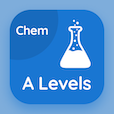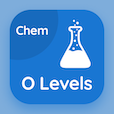Cambridge Online Courses (GCE)

A Level Chemistry MCQs

A Level Chemistry MCQ - Topics

Learn Rate Equations Multiple Choice Questions (MCQ Quiz), Rate Equations quiz answers PDF to learn a level chemistry course for a level chemistry online classes. Reaction Kinetics Multiple Choice Questions and Answers (MCQs), Rate Equations quiz questions for GRE prep classes. Rate Equations Book PDF: rate of reaction, rate equations, order of reaction, catalysts test prep for 2 year online degrees.

"The order of the reaction is the power to which concentration of reactant is" MCQ PDF: rate equations App APK with lowered, raised, stopped, and constant choices for GRE prep classes. Study rate equations quiz questions for merit scholarship test and certificate programs for free online classes.

## MCQs on Rate Equations Quiz

MCQ: The order of the reaction is the power to which concentration of reactant is

lowered
raised
stopped
constant

MCQ: The order of reaction are whole numbers

always
seldom
forever
never

MCQ: The rate constant is the

proportionality constant
K
kΔH
none of above

MCQ: The effect of concentration of reagent on the rate of reaction determines

order of reaction
concentration of products
concentration of reactants
energy of activation

MCQ: The overall representation of a reaction rate in terms of the equation is known as

rate of reaction
rate equation
reaction equation
balanced equation

### More Quizzes on A Level Chemistry Book